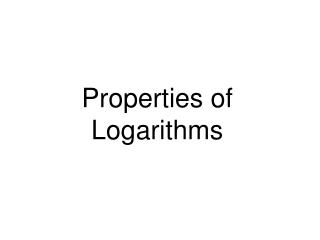# Properties of Logarithms - PowerPoint PPT PresentationDownload PresentationProperties of Logarithms

Properties of Logarithms
Download Presentation## Properties of Logarithms

- - - - - - - - - - - - - - - - - - - - - - - - - - - E N D - - - - - - - - - - - - - - - - - - - - - - - - - - -
##### Presentation Transcript

1. Properties of Logarithms

2. The Product Rule Let b, M, and N be positive real numbers with b 1. logb (MN) = logbM + logbN The logarithm of a product is the sum of the logarithms. For example, we can use the product rule to expand ln (4x): ln (4x) = ln 4 + ln x.

3. æ ö M log ç ÷ = log M - log N è ø b N b b The Quotient Rule Let b, M and N be positive real numbers with b  1. The logarithm of a quotient is the difference of the logarithms.

4. The Power Rule Let b, M, and N be positive real numbers with b = 1, and let p be any real number. log bM p = p log bM The logarithm of a number with an exponent is the product of the exponent and the logarithm of that number.

5. Solution a. log4 2 + log4 32 = log4 (2 • 32) Use the product rule. = log4 64 Although we have a single logarithm, we can simplify since 43 = 64. = 3 Text Example Write as a single logarithm: a. log4 2 + log4 32

6. Problems Write the following as single logarithms:

7. The Change-of-Base Property For any logarithmic bases a and b, and any positive number M, The logarithm of M with base b is equal to the logarithm of M with any new base divided by the logarithm of b with that new base.

8. Solution Because Solution Because This means that This means that Example: Changing Base to Common Logs Use common logarithms to evaluate log5 140. Example: Changing Base to Natural Logs Use natural logarithms to evaluate log5 140.

9. Use logarithms to evaluate log37. Solution: Example or so